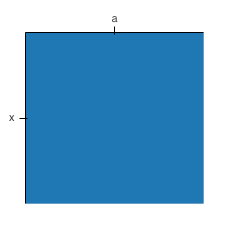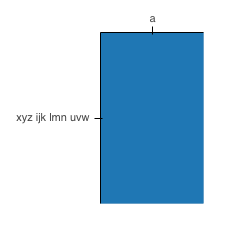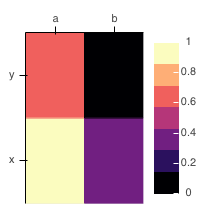# Unable to fix plotting area aspect ratio

Hello,

I am trying to plot a matrix with `rect` glyphs. However, my data varies in length of rows and columns and length of axes labels. I have discovered that the plot aspect ratio is mainly affected by the label size:

``````from bokeh.plotting import figure
from bokeh.plotting import show

fig = figure(
x_axis_location="above",
x_range=["a"],
y_range=["x"],
toolbar_location=None,
)

fig.rect(
'x',
'y',
1.0,
1.0,
source={"x": [0.5], "y": [0.5]},
)

fig.plot_width = 200
fig.plot_height = 200

show(fig)
``````

Which produces a square of aspect ratio 1:However, if I change an axis label length to `y_range=["xyz ijk lmn uvw"]`, the I get a distorted square:even if I set `fig.aspect_ratio = 1.0`.

Another frustrating case is when I include a colorbar because it also produces a distorted (i.e. not square) plot:

``````from bokeh import palettes
from bokeh.models import LinearColorMapper, ColorBar
from bokeh.plotting import figure
from bokeh.plotting import show
from bokeh.transform import transform

fig = figure(
x_axis_location="above",
x_range=["a", "b"],
y_range=["x", "y"],
toolbar_location=None,
)

colormapper = LinearColorMapper(palette=palettes.magma(7))

fig.rect(
'x',
'y',
1.0,
1.0,
source={
"x": [0.5, 0.5, 1.5, 1.5],
"y": [0.5, 1.5, 0.5, 1.5],
"c": [1.0, 0.6, 0.3, 0.0],
},
color=transform("c", colormapper),
)

color_bar = ColorBar(
color_mapper=colormapper,
location=(0, 0),
)

fig.plot_width = 200
fig.plot_height = 200
fig.aspect_ratio = 1

fig.add_layout(color_bar, 'right')
``````Futhermore, the `inner_width` and `inner_height` attributes of `fig` are set to `None` and are read only.

Is there a way of ensuring the aspect ratio is respected, regardless of the axis label sizes or presence of a colorbar?

I’m using Bokeh 2.2.3 on python 3.8.

HI @pabloesteban first thing to mention is that there is an in-progress PR to afford wrapping for tick labels

So in the near future that should help ameliorate these situations somewhat.

As to the question at hand, the `match_aspect` options are only compatible with automatic data-ranges, which categorical ranges are not. So they will not be helpful here. The best control you have right now is the `min_border` options:

You can control:

• the overall canvas size (`plot_width`, etc)
• the minimum border around the inner plot area

So, if you make the min border values large enough to accommodate the largest tick label you will ever see, then that area will not grow or shrink if labels change, and you you can effectively (but indirectly) control the dimensions of the inner plot area (e.g. to make it have the aspect ratio to desire).

Thank you @Bryan. I’ll give it a shot.

1 Like